# Jordan measure

Jump to: navigation, search

of a parallelepiped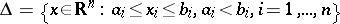inThe volume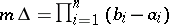of this parallelepiped. The following are defined for a bounded set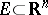: the outer Jordan measure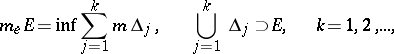and the inner Jordan measurewhere the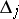are pairwise disjoint (here theare parallelepipeds of the form ). A setis said to be Jordan measurable (squarable for, cubable for) if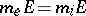or, equivalently, if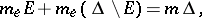where. In this case, the Jordan measure is. A bounded setis Jordan measurable if and only if its boundary has Jordan measure zero (or, equivalently, if its boundary has Lebesgue measure zero).

The concept of this measure was introduced by G. Peano  and C. Jordan . The outer measure is the same forand(the closure of, cf. Closure of a set) and is equal to the Borel measure of. The Jordan-measurable sets form a ring of sets on which the Jordan measure is a finitely-additive function. See also Squarability.